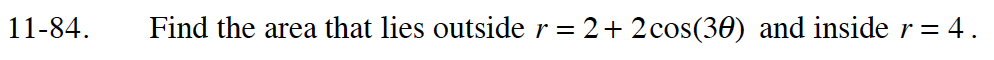### Home > CALC > Chapter 11 > Lesson 11.2.4 > Problem11-84

11-84.

Find the area that lies outside r = 2 + 2cos(3θ) and inside r = 4. Homework Help ✎r = 4 is a circle with a radius of 4.
Subtract the area inside of r = 2 + 2cos(3θ) from the area of the circle.

The area inside of one "petal" is: